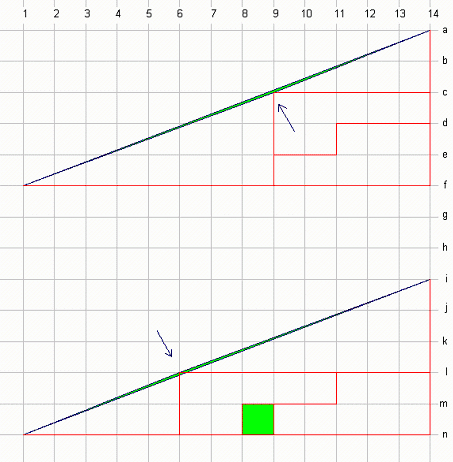GeometryIf you look carefully, you can see that the pieces do not line up on the larger triangle.  The big triangle has a height/length ratio of  5/13 (0.3846153).
On the top picture: the left sub-triangle is 3/8 (0.375) and the right sub-triangle is 2/5 (0.4).  The green diamond-shaped wedge is the area deficit from the true hypontenuse.  When the pieces are switched on the bottom picture, the green wedge is now the area excess from the true hypontenuse.

Area of big triangle = 0.5*5*13 = 32.5
The area of individual top pieces = 15 + (0.5*2*5) + (0.5*3*8) = 32
The area of the individual bottom pieces + green square = 33
The area of the wedge on top + area of wedge on bottom = 1.  The wedge for each triangle is 0.5